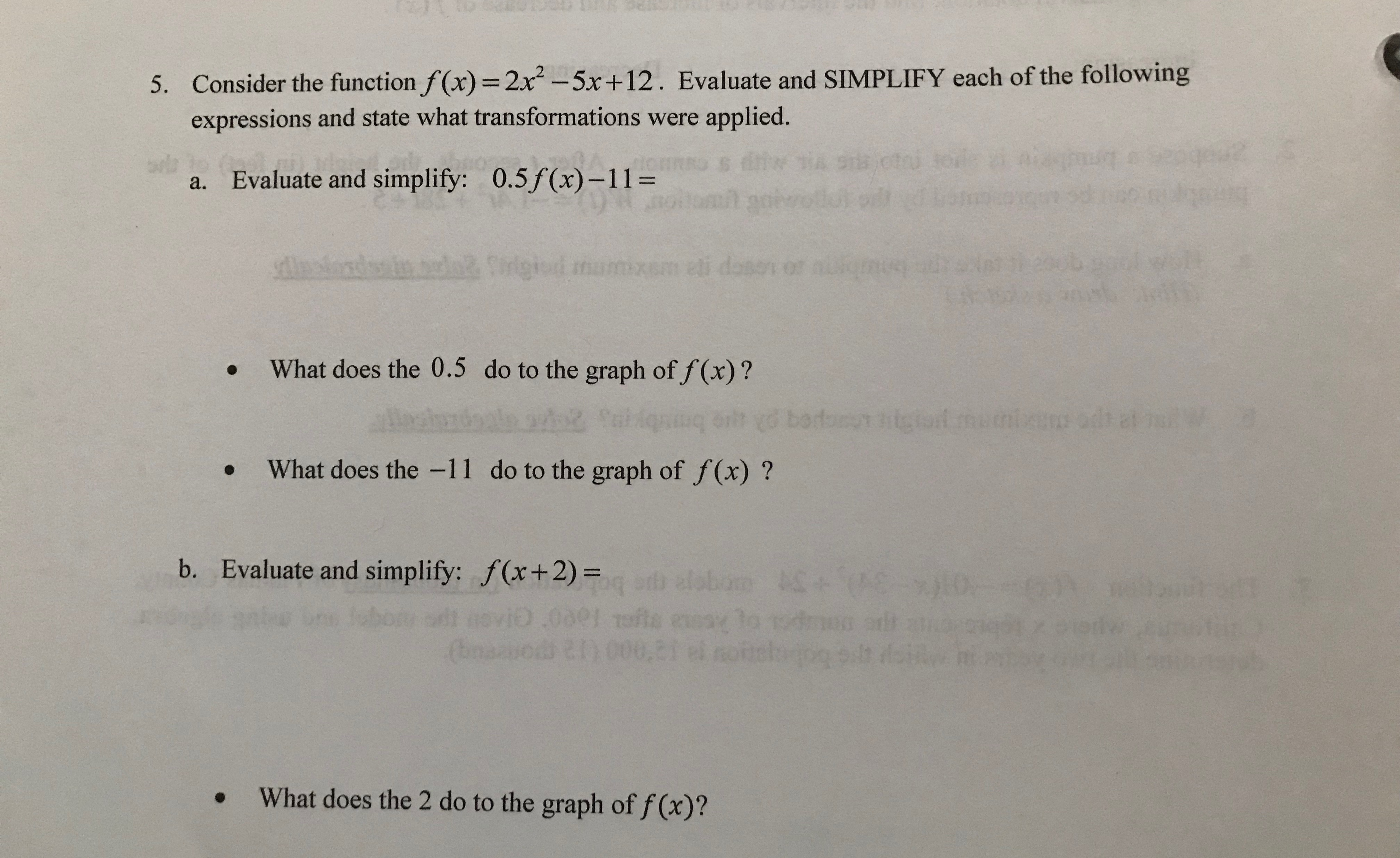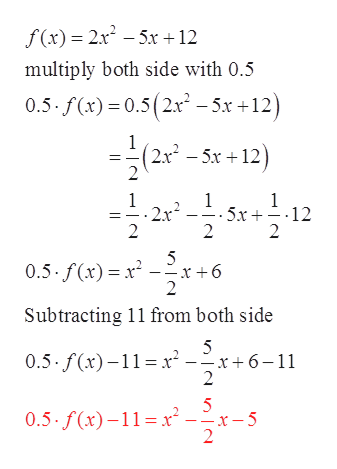# 5. Consider the function f(x) = 2x-5x+12. Evaluate and SIMPLIFY each of the followingexpressions and state what transformations were applied.A9TCSaa.Evaluate and simplify: 0.5 f(x)-11=Sgrseli dedabnds108P NWhat does the 0.5 do to the graph of f(x)?2.eOWhat does the -11 do to the graph of f(x) ?b. Evaluate and simplify: f(x+ 2) =4\$HIE(na21What does the 2 do to the graph of f(x)?

Question
174 viewshelp_outlineImage Transcriptionclose5. Consider the function f(x) = 2x-5x+12. Evaluate and SIMPLIFY each of the following expressions and state what transformations were applied. A9TC Sa a. Evaluate and simplify: 0.5 f(x)-11= Sgrs eli de dabnds 108P N What does the 0.5 do to the graph of f(x)? 2.e O What does the -11 do to the graph of f(x) ? b. Evaluate and simplify: f(x+ 2) = 4\$H IE (na 21 What does the 2 do to the graph of f(x)? fullscreen
check_circle

Step 1

Consider the below function

Step 2

Part a.

In order to evaluate 0.5f(x) – 11, first multiply the function f(x) with 0.5 and then subtract 11 from it.help_outlineImage Transcriptionclose2x25x + 12 f(x) multiply both side with 0.5 0.5- f(x) 0.5(2x -5x +12) 1 2x2-5x12 2 1 - 2x 2 1 5x 12 2 _ 2 5 0.5. f(x) x x+6 2 Subtracting 11 from both side 5 0.5. f(x)11 x--x+6-11 2 5 0.5. f(x)-11 x 2 5 fullscreen
Step 3

Multiplying a value less then 1 to a function always compresses the function vertically and subtracting a value from the function always vertically translate it down.

Therefore,

Multi...

### Want to see the full answer?

See Solution

#### Want to see this answer and more?

Solutions are written by subject experts who are available 24/7. Questions are typically answered within 1 hour.*

See Solution
*Response times may vary by subject and question.
Tagged in

### Applications of Mathematics